# Sets in LISP

• Last Updated : 31 Jan, 2022

A set is an unordered collection of items. A set is just like other data structures. In C++ we have a similar data structure called a hash map. Common lisp does not provide a built-in set data type, but it provides a number of functions that allow set operations to be performed onto a list. Using them, we can add and remove items from the list and also check if an item is present in the list. We can also perform union, intersection, and difference operations on the list.

### Implementing  Sets in the Lisp:

Sets are implemented using the adjoin function. Adjoin function allows us to build up a set.  Adjoin function can take a list as an argument and returns a set. The adjoin function is defined as follows:

Syntax:

`adjoin item list &key key test  => new-list`

Here, the item is an object, the list is a proper list, the test is a designator for a function of two-argument and it returns a boolean value, the key is a designator function for a function of one argument.

These key and test argument is used for checking whether an item is present in the list or not. If the item is present in the list, then the item is not added to the set. If the item is not present in the list, then the item is added to the set. We can use the macro pushnew to add an item to the set, if the item is not present in the set, and DELETE or REMOVE to remove or delete the item.

Example:

Create a new lisp file and save it as set.lisp

## Lisp

 `;; Implementing the Sets in the Lisp`` ` `(defparameter ``*``mynewset``*` `()) ;; empty set``(adjoin ``1` `*``mynewset``*``) ;; ``*``mynewset``*` `is now (``1``)``(adjoin ``2` `*``mynewset``*``) ;; ``*``mynewset``*` `is now (``1` `2``)`` ` `; adjoin did ``not` `change the original set``; so it remains same``(write ``*``mynewset``*``) ;; (``1` `2``)``(terpri) ;; prints a new line``(``setf` `*``mynewset``*` `(adjoin ``1` `*``mynewset``*``)) ;; ``*``mynewset``*` `is now (``1``)``(``setf` `*``mynewset``*` `(adjoin ``2` `*``mynewset``*``)) ;; ``*``mynewset``*` `is now (``1` `2``)`` ` `; adding an existing value``(pushnew ``2` `*``mynewset``*``) ;; ``*``mynewset``*` `is now (``1` `2``)`` ` `; no duplicate allowed``(write ``*``mynewset``*``) ;; (``1` `2``)``(terpri) ;; prints a new line`` ` `; pushing a new value``(pushnew ``3` `*``mynewset``*``) ;; ``*``mynewset``*` `is now (``1` `2` `3``)``(write ``*``mynewset``*``) ;; (``1` `2` `3``)``(terpri) ;; prints a new line`

Output: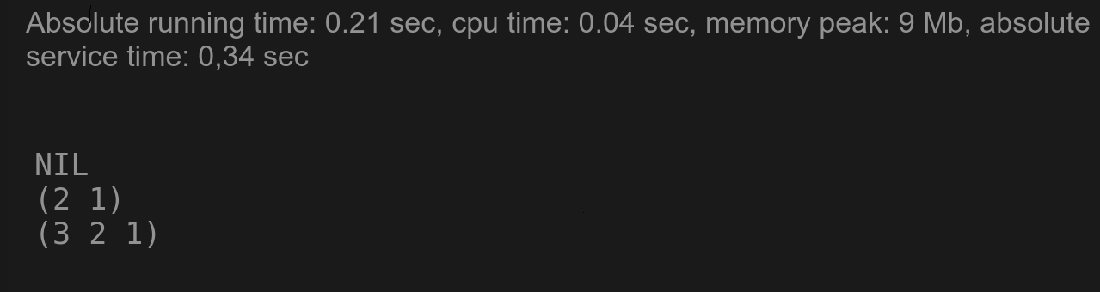Set implementation

### Checking Membership in a Set:

The Membership is a function that checks whether an item is present in the set or not. The membership function is defined as follows:

member item list &key :test :test-not :key

member-if predicate list &key :key

member-if-not predicate list &key :key

These functions probe the list for the item. If the item is present in the list, then the membership function returns t. If the item is not present in the list, then the membership function returns nil. The search is done at the top level only. The search is done in the order of the list.

Syntax:

`member item list test &key key &key => tail or nil`

Here, the item is an item that is to be found, the list is the list to be searched, the test is the item to be compared, and &key is the function key. Key is a function for extracting the value before the test. Member function searches the list for the item. If the item is present in the list, then the membership function returns t. If the item is not present in the list, then the membership function returns nil. The search is done at the top level only. The search is done in the order of the list.

Example:

Create a new lisp file and save it as membership.lisp.

## Lisp

 `;; Checking Membership in a Set`` ` `(write (``member` `0` `(``list` `1` `2` `3``)))  ;; returns ``nil``(terpri) ;; prints a new line`` ` `(write (``member``-``if` `#'evenp '(5 7 9 8 4 ) ));; returns t``(terpri) ;; prints a new line`` ` `(write (``member``-``if``-``not`  `#'numberp '(3 7 2 5/3 'not 'number ))) ;; returns nil`

Output: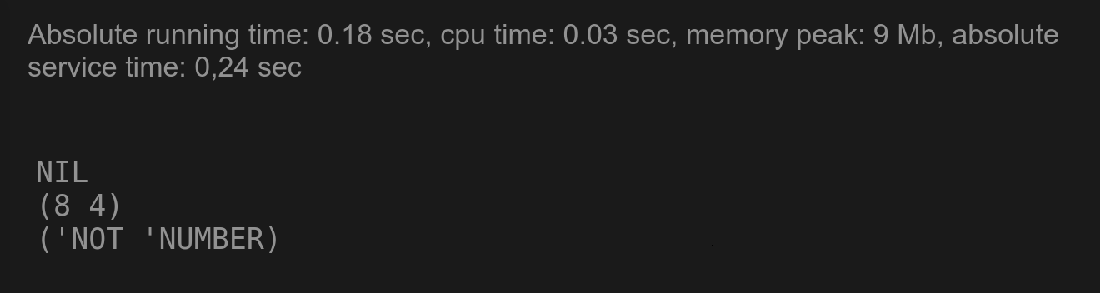Membership in Set

### Set union in Lisp:

The union means the set of all the items in the list. Using the union group function, we can find the union of two sets. The union function is defined as follows:

Syntax:

```union list1 list2 &key :test
nunion list1 list2 &key :test  ```

Here, the union function takes two lists, list1 and list2, and returns a new list that contains all the items from both the lists. Duplicate items are not allowed in the new list.

Example:

Create a new lisp file and save it as union.lisp.

## Lisp

 `;; Set union in Lisp`` ` ` ` `(setq set1 (union ``'(1 2 3) '``(``2` `3` `4``))) ;; set1 is (``1` `2` `3` `4``)``(terpri) ;; prints a new line`` ` `(setq set2 (union ``'(a b 5 6 7 f h) '``(``5` `6` `7` `a b g h)))`` ` `(write set1) ;; (``1` `2` `3` `4``)``(terpri)``(write set2) ;; (F ``5` `6` `7` `A B G H)``(terpri)`

Output: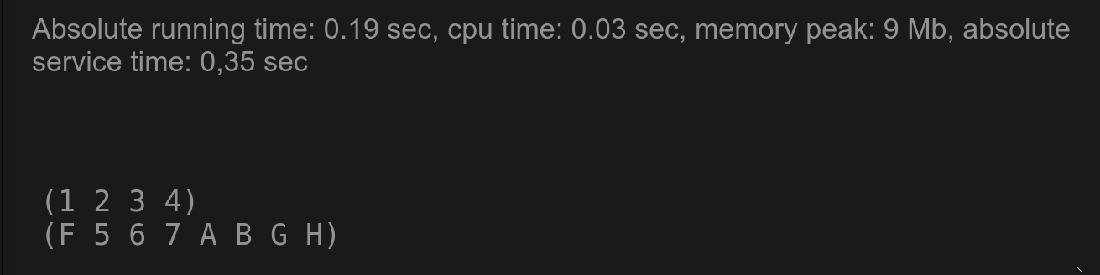Union in Set

### Set intersection in Lisp:

The intersection can think of as the set of items that are common to both sets. To do intersection, we can use the intersection group of functions. It takes two lists and returns a new list that contains all the items that are common to both lists. The intersection function is defined as follows:

Syntax:

```intersection list1 list2 &key :test
nintersection list1 list2 &key :test```

Here the intersection function takes two lists, list1 and list2, and returns a new list that contains all the items that are common to both the lists. The intersection is the same as the intersection function except that it destroys the list1 using its cells to construct the result list2 and returns the result list2.

Example:

Create a new lisp file and save it as intersection.lisp.

## Lisp

 `;; Set intersection in Lisp`` ` ` ` `(setq set1 (intersection ``'(1 2 3) '``(``2` `3` `4``))) ;; set1 is (``2` `3``)``(terpri) ;; prints a new line`` ` `(setq set2 (intersection ``'(a b 5 6 7 f h) '``(``5` `6` `7` `a b g h))) `` ` `(write set1) ;; (``2` `3``)``(terpri) ;; prints a new line``  ` `( write set2) ;; (A B ``5` `6` `7` `H)``(terpri)  ;; prints a new line`

Output: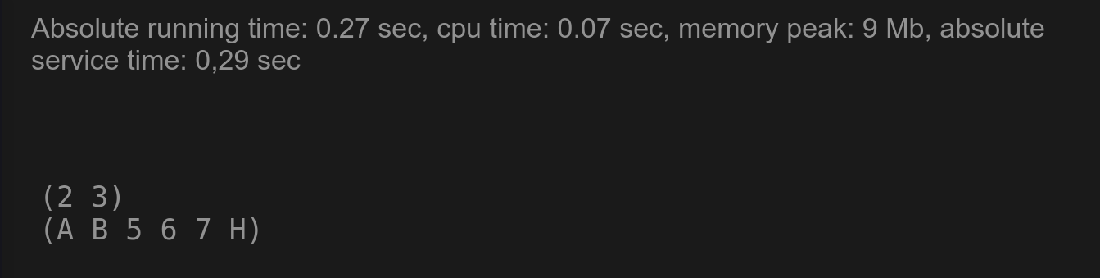A set intersection in Lisp

### Set Difference in Lisp:

A set difference is the set of items that are in the first set but not in the second set. Using the set difference function, we can find the set difference of two sets. The set difference function is defined as follows:

Syntax:

```set-difference list1 list2 &key :test
nset-difference list1 list2 &key :test```

Here, the set difference function takes two lists, list1 and list2, and returns a new list that contains all the items that are in the first list but not in the second list. The nset-difference is the same as the set difference function except that it destroys the list1 using its cells to construct the result list2 and returns the result list2.

Set-difference returns a list of items that are in the first list but not in the second list.

Nset is the destructive version of the set-difference, it destroys the list1 and returns the result list2.

Example:

Create a new lisp file and save it as set-difference.lisp.

## Lisp

 `;; Set Difference in Lisp`` ` ` ` `(write(setq lst1 (``list` `"A"` `"b"` `"C"` `"d"``) lst2 (``list` `"a"` `"B"` `"C"` `"d"``))) ``;; lst1 is (``"A"` `"b"` `"C"` `"d"``) lst2 is (``"a"` `"b"` `"C"` `"d"``)``(write(set``-``difference lst1 lst2)) ;; returns (``"A"` `"C"``)``(terpri) ;; prints a new line`` ` `(write(set``-``difference lst1 lst2 :test '``equal``)) ;; returns (``"A"` `"C"``)``(terpri) ;; prints a new line`` ` `(write(nset``-``difference lst1 lst2 :test ``#'string=));; returns ("A" "b" "C" "d")``(terpri) ;; prints a new line`

Output: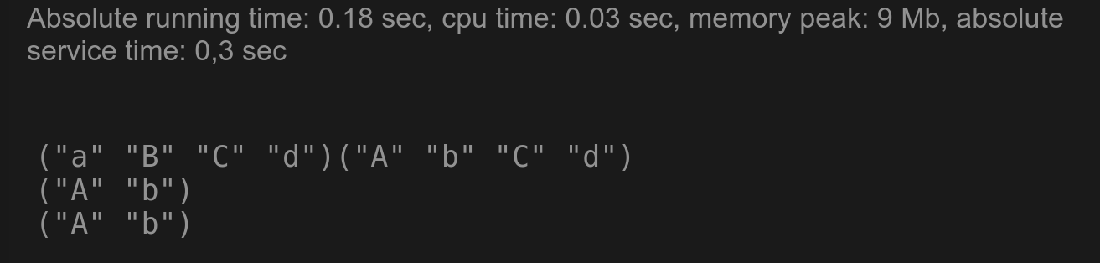Set difference in lisp

My Personal Notes arrow_drop_up